Courses

# Production

## 45 Questions MCQ Test GATE Mechanical (ME) 2022 Mock Test Series | Production

Description
This mock test of Production for GATE helps you for every GATE entrance exam. This contains 45 Multiple Choice Questions for GATE Production (mcq) to study with solutions a complete question bank. The solved questions answers in this Production quiz give you a good mix of easy questions and tough questions. GATE students definitely take this Production exercise for a better result in the exam. You can find other Production extra questions, long questions & short questions for GATE on EduRev as well by searching above.
QUESTION: 1

### Consider the figure shown below:-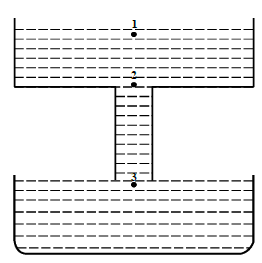Reason for the aspiration effect is the:-

Solution: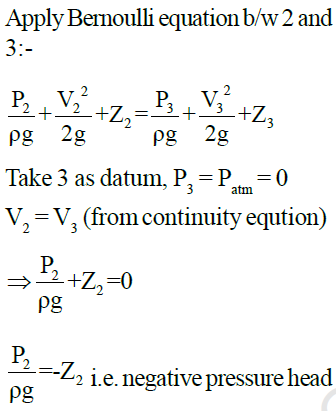QUESTION: 2

### Consider the figure shown below. There is a downsprue of length 400mm has a diameter of 30mm at its top end. Height of 96mm of liquid metal is maintained in pouring cup. If aspiration effect is to be avoided, then diameter of downsprue at its lower end is:-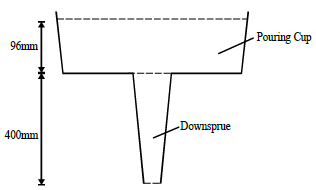Solution: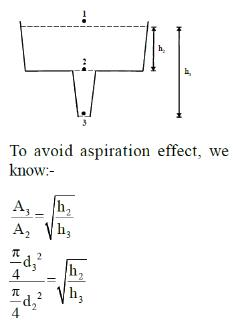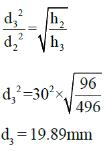QUESTION: 3

### Riser design with its height equal to diameter is used to feed a casting 20cm × 20cm × 0cm. On solidification, volume shrinkage is 3% and volume of riser is 3 times of shrinkage volume. Height of riser is :-

Solution: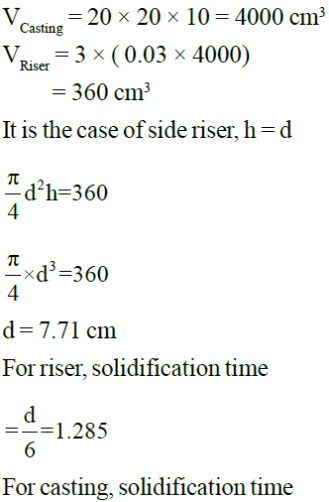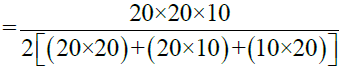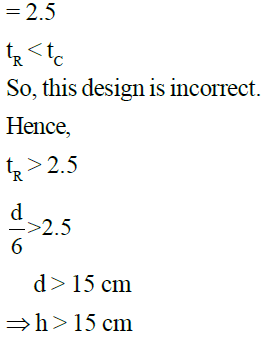QUESTION: 4

Casting with dimensions 50cm × 40cm × 10cm size solidifies in 20min. The solidification time for 40cm × 30cm × 5cm casting under similar condition is:-

Solution: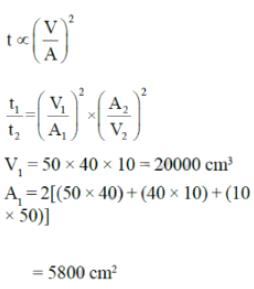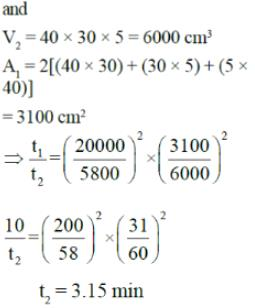QUESTION: 5

A sprue for avoiding aspiration to deliver liquid iron at a rate of 20 kg/s. The density of iron is 7800 kg/m3. The height of pouring basin is 9cm. and height of sprue is 25cm. Diameter of sprue base is:-

Solution: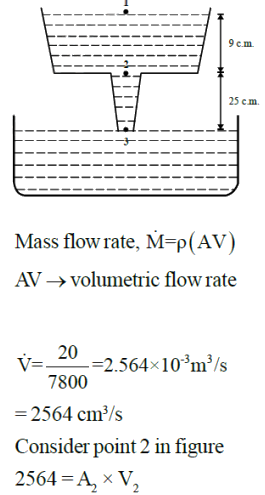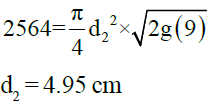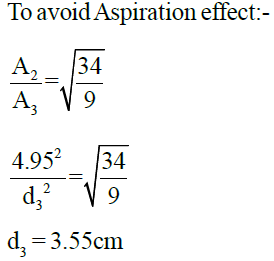QUESTION: 6

Cube of side ‘a’ and solid sphere of diameter ‘d’ are made from same material. Ratio of their solidification time is :-;

Solution: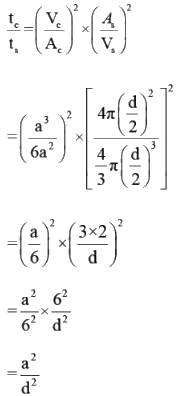QUESTION: 7

Shrinkage allowance is made up by :-

Solution:

When a hollow cylinder of internal diameter d1 and external diameter d2 is to be cast, then shrinkage allowance is subtracted from d1 and added to d2.

QUESTION: 8

Which of the following are the requirements of an ideal gating system?

1. Molten metal should enter mould cavity with as high velocity as possible.

2. It should facilitate complete filling of mould cavity.

3. It should be able to prevent absorption of air or gases from the surroundings by molten metal while flowing through it.

Select the correct answer using codes given below:-

Solution:
QUESTION: 9

Match the following contents of green sand in Group1 with their approximate percentage in Group2

1. Silica                A. 5-10%
2. Clay                 B. 2-5%
3. Bentonite          C. 8-15%
4. Coal Dust          D. 7-8%
5. Water                E. upto 75%

Solution:
QUESTION: 10

Correctly match Group-I and Group-II

Group-I
P. Coal Dust
Q. Dextrin & Molasses
R. Al2O3
S. Wood Flour

Group-II
E. Mould Hardness
F. Hot Strength
G. Surface Finish
H. Collapsibility

Solution:
QUESTION: 11

Common data for 11 & 12

A square plate [60mm × 60mm] is to be cut from a sheet of 1.5mm thickness. Shear strength of the
sheet material is 240 MPa

Q. Size of the punch and the die respectively are

Solution: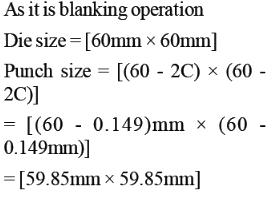QUESTION: 12

Common data for 11 & 12

A square plate [60mm × 60mm] is to be cut from a sheet of 1.5mm thickness. Shear strength of the sheet material is 240 MPa

Q. If cutting is complete at 60% penetration of the punch. Suitable shear angle for the punch to bring the work within the capacity of 38880 kN is

Solution: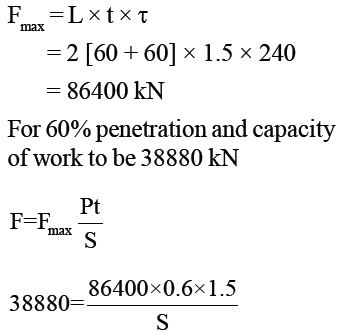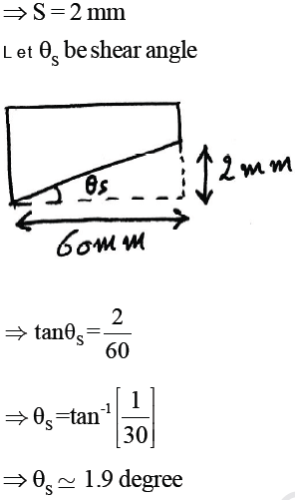QUESTION: 13

Barelling is a term associated with which of the following processes

Solution:
QUESTION: 14

If A0 is the initial cross-sectional area of the billet and Af, final crosssectional area of the extruded part, then the reduction ratio is expressed as

Solution:

Extrusions ratio is the ratio of the original cross-sectional area of the starting billet to the final cross-sectional area of the extruded part and it is given by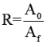*Answer can only contain numeric values
QUESTION: 15

In a rolling operation of mild steel plate, in a single pass the final thickness is reduced to two third of initial thickness. The entrance speed is 14 m/min and roll diameter is 450mm. If the plate width is increased by 2.5% during the operation. The exit velocity is ___ m/min.

(Important - Enter only the numerical value in the answer)

Solution: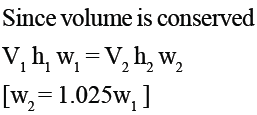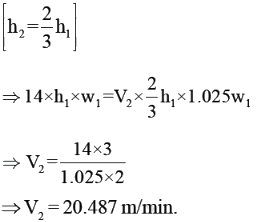QUESTION: 16

In rolling operation, the power required varies.

1) Directly proportional to the roll gap

2) Inversely proportional to the roll gap

3) Directly proportional to the roll force

4) Directly proportional to roll speed

Which of the statements are true

Solution: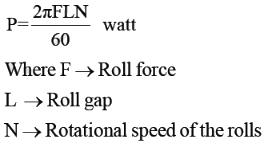QUESTION: 17

Which one of the following is a heat treatment process for surface hardening?

Solution:
QUESTION: 18

A copper strip of 200mm width and 30mm thickness is to be rolled to a thickness of 25mm. The roll of radius 300mm rotates at 100rpm. The average shear strength of the work material is 180Mpa. The roll strip contact length and the roll force are

Solution:
QUESTION: 19

A solid cylinder of diameter 1000mm and height 50mm is forged between two friction-less flat dies to a height of 25 mm.
The percentage change in diameter is

Solution: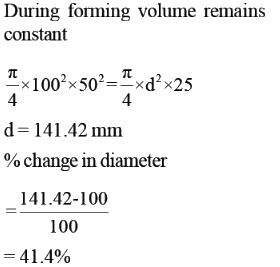QUESTION: 20

Using direct extrusion process, a round billet of 100mm length and 50mm diameter is extruded. Considering an ideal deformation process (no friction and no redundant work) extrusion ratio 4 and average flow stress of material 300 MPa, the Pressure (MPa) on the ram will be

Solution: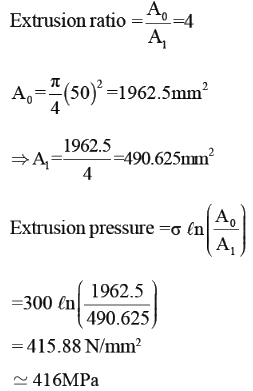QUESTION: 21

Which one of the following statements regarding the formation of continuous chips is incorrect?

Solution:

Large rake angle and high cutting speeds are desirable for formation of continuous chips.

QUESTION: 22

Which of the following statements is true about nose radius?

Solution:
QUESTION: 23

14-A drill bit of 20mm diameter rotating at 500 rpm with a feed rate of 0.2 mm/revolution is used to drill a through hole in Mild Steel plate of 20mm thickness. The depth of cut in this drilling operation is

Solution:
QUESTION: 24

Common data for 24 & 25

During orthogonal cutting process, following data have been observed:-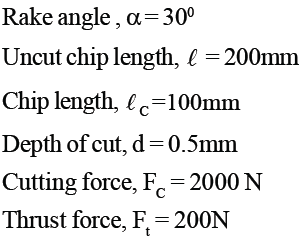Q. Value of shear plane angle is:-

Solution:
QUESTION: 25

Common data for 24 & 25
During orthogonal cutting process, following data have been observed:-Q. Friction angle is given by:-

Solution: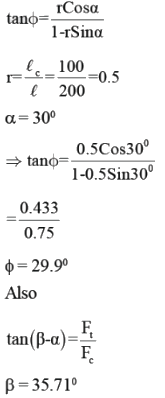QUESTION: 26

In orthogonal cutting operation, rake angle is 150 and coefficient of friction is 0.5. Using Merchant’s theory, that will be the percentage change in chip thickness if friction is doubled?

Solution: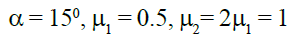QUESTION: 27

In orthogonal cutting of MS tube with HSS tool, cutting speed and rake angle are 15m/min and 150. Chip thickness ratio is 0.35. Friction force and coefficient of friction are 470N and 0.6 respectively. Value of cutting force is:-

Solution: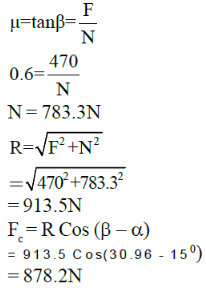QUESTION: 28

Which of the following is correct regarding Built-up Edge?

Solution:
QUESTION: 29

A carbide tool workpiece was found to have life of 3 hours while cutting mild steel workpiece, at the speed of 60m/min. The Taylor’s exponent used be n = 0.3. If the same tool is used at speed 30% higher than the previous one, then the new tool life will be

Solution:QUESTION: 30

Which of the following statements is correct:-

Solution:
QUESTION: 31

Which of the following welding processes results in least heat affected zone near the weld bead

Solution:

In electron beam welding, as the electron beam is concentrated form of energy, HAZ is minimum.

QUESTION: 32

Consider the following statements

I) Strength of weld bead is greater than the strength of parent metal.

II) By increasing the welding speed, heat affected zone increases.

III) At the centre of weld pool and towards the heat affected zone the grain structure is coarser and finer
respectively

IV) As the carbon percentage in steels increases, the weldability is increased.

Which of the above statements is/are correct

Solution:

By increasing welding speed, heat affected zone decreases as parent metal is sunjected to high temperature for less time.

At the centre of weldpool due to low cooling rate coarser grain structure will be produced and towards the heat affected zone due
to higher cooling rate finer grain structure of weld bead will be produced.

Weldability
Mild Steel > Medium Carbon steel
> High Carbon Steel > Alluminium

QUESTION: 33

TIG welding is specificaly used to weld alluminium alloys because:

Solution:

TIG welding setup was specificaly invented to weld alluminium alloy because due to oxide layer formation the weldability of Al is
very poor.
To weld Al workpiece is connected with negative terminal and electode is connected to positive terminal and as electrons are coming out from negative terminal (workpiece), the oxide layer over
the workpiece will peel off.

QUESTION: 34

Consider the following statements

I) Brazing takes place below 4500C

II) Zinc chloride is used as flux in soldering

III) Brass is used as a filler material in brazing

IV) Main principle in soldering and brazing is capillary action.

Which of the statements is/are correct

Solution: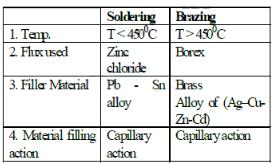QUESTION: 35

To control the heat treatment of the weld bead in arc weldig which of following is helpful.

Solution:

Since flux has lower density as compared to liquid metal, it will float and forms a layer of slag over the bead. The slag coating has three important functions.

1) It protects the bead from attack of atmospheric gases

2) It controls the heat treatment of bead

3) It provides alloys to bead.

*Answer can only contain numeric values
QUESTION: 36

Two rods of diameter 50mm each are joined by flash butt welding. At interface 1.5mm of material melt from each rod which has resistance of 38.6?. If the melting energy is 54 MJ/m3 and and the time required
for melting is 8 seconds. The supply voltage for the process is _____ volts

(Important - Enter only the numerical value in the answer)

Solution: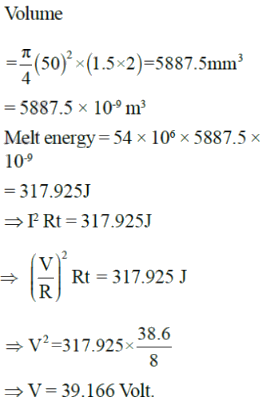QUESTION: 37

A calorically perfect gas (specific heat at constant pressure 1000 J/kg.K) enters and leaves a gas turbine with the same velocity. The temperatures of the gas at turbine entry and exit are 1100 K and 400 K. respectively. The power produced is 4.6 MW and heat escapes at the rate of 300 kJ/s through the turbine casing. The mass flow rate of the gas (in kg/s) through the turbine is.

Solution:
*Answer can only contain numeric values
QUESTION: 38

In an arc welding process the melting efficiency and heat transfer efficiency are 45% and 75% respectively. The area of the weld crossection is 6mm2 and unit energy required to melt the material is 12J/mm3. If welding power is 2.5 kW.
The welding speed is _____mm/s

(Important - Enter only the numerical value in the answer)

Solution: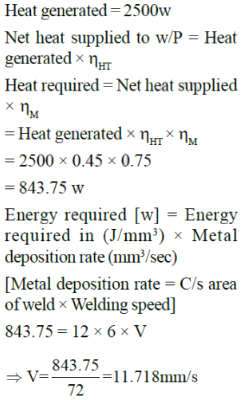*Answer can only contain numeric values
QUESTION: 39

If the thermal efficiency of the process is 65%. The net heat input per unit length of weld is ___kJ/cm

(Important - Enter only the numerical value in the answer)

Solution:
*Answer can only contain numeric values
QUESTION: 40

The crossection area of the weld is _____mm2.

(Important - Enter only the numerical value in the answer)

Solution: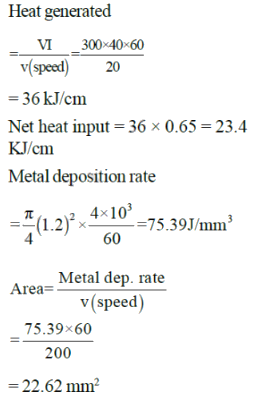QUESTION: 41

During the execution of a CNC part program block N010 G00 X45.0 Y20.0. The type of tool motion will be

Solution:
QUESTION: 42

The following CNC part program block is written to create an arc N010 G20 G91 X5 Y-5 IO J5. The arc radius is

Solution:
QUESTION: 43

Which among the NC operations given below are PRP control maching? TIG Welding (TW), Turning (T), Drilling (D) Punching in sheet Metal (P), Laser cutting of sheet metal (LC) Spot Welding (SW)

Solution:
QUESTION: 44

The tool of an NC machine has to move along a circular arc from (5, 5) to (10, 10) while performing an operation. The center of the arc is at (10, 5). Which one of the following NC tool path commands performs the above mentioned operation?

Solution:
QUESTION: 45

Match the following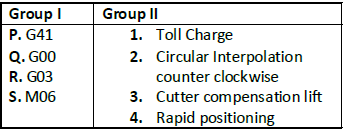Solution: